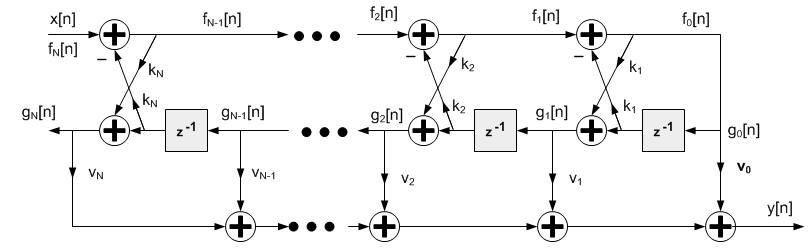Project Ne10 An open, optimized software library for the ARM architecture.
Infinite Impulse Response (IIR) Lattice Filters

## Functions

ne10_result_t ne10_iir_lattice_init_float (ne10_iir_lattice_instance_f32_t *S, ne10_uint16_t numStages, ne10_float32_t *pkCoeffs, ne10_float32_t *pvCoeffs, ne10_float32_t *pState, ne10_uint32_t blockSize)
Initialization function for the floating-point IIR lattice filter. More...

void ne10_iir_lattice_float_c (const ne10_iir_lattice_instance_f32_t *S, ne10_float32_t *pSrc, ne10_float32_t *pDst, ne10_uint32_t blockSize)
Specific implementation of ne10_iir_lattice_float using plain C. More...

void ne10_iir_lattice_float_neon (const ne10_iir_lattice_instance_f32_t *S, ne10_float32_t *pSrc, ne10_float32_t *pDst, ne10_uint32_t blockSize) asm("ne10_iir_lattice_float_neon")
Specific implementation of ne10_iir_lattice_float using NEON SIMD capabilities. More...

## Variables

void(* ne10_iir_lattice_float )(const ne10_iir_lattice_instance_f32_t *S, ne10_float32_t *pSrc, ne10_float32_t *pDst, ne10_uint32_t blockSize)
Processing function for the floating-point IIR lattice filter. More...

## Detailed Description

This set of functions implements lattice filters for and floating-point data types. Lattice filters are used in a variety of adaptive filter applications. The filter structure has feedforward and feedback components and the net impulse response is infinite length. The functions operate on blocks of input and output data and each call to the function processes `blockSize` samples through the filter. `pSrc` and `pDst` point to input and output arrays containing `blockSize` values.
Algorithm:Infinite Impulse Response Lattice filter
```     fN(n)   =  x(n)
fm-1(n) = fm(n) - km * gm-1(n-1)   for m = N, N-1, ...1
gm(n)   = km * fm-1(n) + gm-1(n-1) for m = N, N-1, ...1
y(n)    = vN * gN(n) + vN-1 * gN-1(n) + ...+ v0 * g0(n)
```
`pkCoeffs` points to array of reflection coefficients of size `numStages`. Reflection coefficients are stored in time-reversed order.
```     {kN, kN-1, ....k1}
```
`pvCoeffs` points to the array of ladder coefficients of size `(numStages+1)`. Ladder coefficients are stored in time-reversed order.
```     v0, v1, ...vN
```
`pState` points to a state array of size `numStages + blockSize`. The state variables shown in the figure above (the g values) are stored in the `pState` array. The state variables are updated after each block of data is processed; the coefficients are untouched.
Instance Structure
The coefficients and state variables for a filter are stored together in an instance data structure. A separate instance structure must be defined for each filter. Coefficient arrays may be shared among several instances while state variable arrays cannot be shared. There are separate instance structure declarations for each of the 3 supported data types.
Initialization Functions
There is also an associated initialization function for each data type. The initialization function performs the following operations:
• Sets the values of the internal structure fields.
• Zeros out the values in the state buffer.
Use of the initialization function is optional. However, if the initialization function is used, then the instance structure cannot be placed into a const data section. To place an instance structure into a const data section, the instance structure must be manually initialized. Set the values in the state buffer to zeros and then manually initialize the instance structure as follows:
```*arm_iir_lattice_instance_f32 S = {numStages, pState, pkCoeffs, pvCoeffs};
```
where `numStages` is the number of stages in the filter; `pState` points to the state buffer array; `pkCoeffs` points to array of the reflection coefficients; `pvCoeffs` points to the array of ladder coefficients.
Fixed-Point Behavior
Care must be taken when using the fixed-point versions of the IIR lattice filter functions. In particular, the overflow and saturation behavior of the accumulator used in each function must be considered. Refer to the function specific documentation below for usage guidelines.

## Function Documentation

 void ne10_iir_lattice_float_c ( const ne10_iir_lattice_instance_f32_t * S, ne10_float32_t * pSrc, ne10_float32_t * pDst, ne10_uint32_t blockSize )

Specific implementation of ne10_iir_lattice_float using plain C.

Definition at line 112 of file NE10_iir.c.

 void ne10_iir_lattice_float_neon ( const ne10_iir_lattice_instance_f32_t * S, ne10_float32_t * pSrc, ne10_float32_t * pDst, ne10_uint32_t blockSize )

Specific implementation of ne10_iir_lattice_float using NEON SIMD capabilities.

 ne10_result_t ne10_iir_lattice_init_float ( ne10_iir_lattice_instance_f32_t * S, ne10_uint16_t numStages, ne10_float32_t * pkCoeffs, ne10_float32_t * pvCoeffs, ne10_float32_t * pState, ne10_uint32_t blockSize )

Initialization function for the floating-point IIR lattice filter.

Parameters
 [in] *S points to an instance of the floating-point IIR lattice structure. [in] numStages number of stages in the filter. [in] *pkCoeffs points to the reflection coefficient buffer. The array is of length numStages. [in] *pvCoeffs points to the ladder coefficient buffer. The array is of length numStages+1. [in] *pState points to the state buffer. The array is of length numStages+blockSize. [in] blockSize number of samples to process.

Definition at line 44 of file NE10_iir_init.c.

## Variable Documentation

 void(* ne10_iir_lattice_float) (const ne10_iir_lattice_instance_f32_t *S, ne10_float32_t *pSrc, ne10_float32_t *pDst, ne10_uint32_t blockSize)

Processing function for the floating-point IIR lattice filter.

Parameters
 [in] *S points to an instance of the floating-point IIR lattice structure. [in] *pSrc points to the block of input data. [out] *pDst points to the block of output data. [in] blockSize number of samples to process.

Definition at line 193 of file NE10_init_dsp.c.